## Sunday, September 18, 2016

### Math Practice: Multiply by 3 Practical Math Skills

This math practical test includes scenarios that apply multiplying by the number three. The answer for each scenario includes a picture for counting the total number, how to add, how to multiply, and how to say the math multiplication phrase.

Although there are several different ways to approach multiplying by three, I featured only one approach in order to keep it simple and less confusing. With these examples, I chose adding the number being multiplied by three to itself three times.

After practicing this way and feeling very comfortable with these examples, you may also go through the game and ask for other ways to get the same answer

Directions
1. Click on the first slide to enlarge to full screen.
2. Click on the slide to advance to the next one.
Related Articles - you may wish to review some of these before moving into multiplication
Thanks for visiting my Student Survive 2 Thrive blog. I hope you find my resources to be helpful! Feel free to post comments in the comment section below the slides.Multiply by 3 Practical Math SkillsFree Math Practice Test - Multiplication3 X 3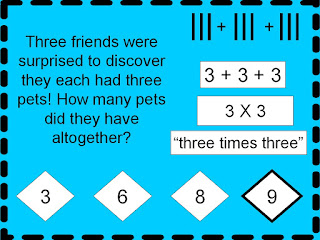3 x 3 = 911 X 3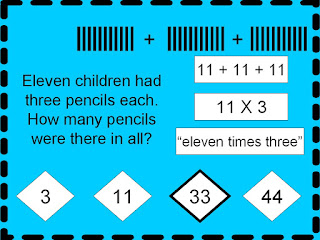11 X 3 = 333 X 03 X 0 = 03 X 83 X 8 = 243 X 53 X 5 = 153 X 123 X 12 = 363 X 73 X 7 = 21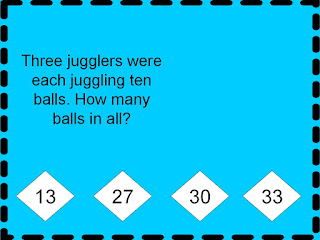3 X 103 X 10 = 303 X 63 X 6 = 18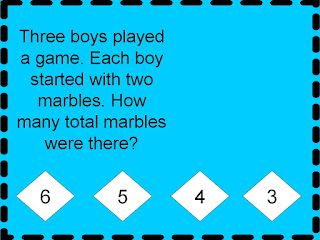3 X 2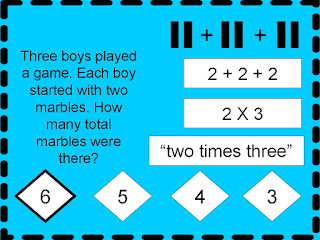3 X 2 = 63 X 93 X 9 = 273 X 13 X 1 = 33 X 43 X 4 = 12Free Math Practice Test at Student Survive 2 Thrive